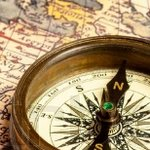# Geography Quiz / US Cities by 3 letter combinations (B--)

Random Geography or Letter Quiz

## Can you name the most populous US city for each 3 letter combination (starting with B)?

#### byRobzle  Plays Quiz not verified by Sporcle

How to Play
Score
0/89
Timer
15:00
Letter combinationCityState (City pop.)
BabNY (12,145)
BaiWA (24,522)
BakCA (380,874)
BalMD (611,648)
BanCA (104,834)
BarCA (92,101)
BasAL (33,202)
BatLA (225,374)
BauMN (23,750)
BaxMN (8,278)
BayFL (111,657)
BbaOH (7,485)
BbcMO (11,334)
BbeLA (12,272)
BbiMN (16,041)
BboTX (253,888)
BbsNM (37,764)
BbuID (14,869)
BeaCA (469,450)
BecWV (16,404)
BedMA (95,120)
BeeIN (14,795)
BelWA (144,444)
BemNC (15,977)
BenIN (102,245)
BerAZ (242,354)
BesAL (26,386)
BetNJ (130,215)
BevMA (41,816)
Letter combinationCityState (City pop.)
BexOH (13,786)
BiaSC (133,114)
BidME (21,488)
BigTX (27,905)
BilAL (190,265)
BinGA (74,854)
BioMI (8,285)
BirAL (210,710)
BisND (72,865)
BixOK (26,724)
BlaMN (64,557)
BleIN (61,882)
BliCA (60,939)
BloCO (111,127)
BluIA (62,316)
BlyCA (19,630)
BoaCO (12,965)
BocTX (253,888)
BodMA (52,987)
BoeTX (16,056)
BogLA (11,789)
BoiID (226,570)
BokNJ (55,131)
BolIL (75,201)
BonFL (56,088)
BooNC (19,205)
BorNC (290,222)
BosMA (685,094)
BotWA (45,533)
BouCO (107,125)
Letter combinationCityState (City pop.)
BowKY (67,067)
BoxSD (9,498)
BoyFL (77,702)
BozMT (46,596)
BraCA (85,396)
BreCA (62,433)
BriCT (146,579)
BroTX (183,299)
BruNJ (57,073)
BryTX (84,021)
BseNJ (8,283)
BsfNY (11,147)
BsoNC (7,096)
BstMO (22,886)
BucAZ (68,453)
BudTX (16,163)
BueCA (110,790)
BufNY (258,612)
BuiCO (6,353)
BulAZ (40,252)
BunME (11,380)
BurPA (302,407)
BuqNM (558,545)
BusOH (879,170)
ButMT (33,901)
BuxME (8,253)
BylNY (12,145)
ByrMS (11,671)
ByvTN (21,532)

## From the Vault See Another

by hjckr

History 60s
If you're not a great speller, you can always lean on phonetics.

### Extras

Created May 10, 2019Source
Tags: